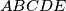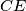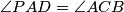### MEMO 2017 pojedinačno problem 3

Kvaliteta:
Avg: 0,0
Težina:
Avg: 6,0

Let$ABCDE$ be a convex pentagon. Let$P$ be the intersection of the lines$CE$ and$BD$. Assume that$\angle PAD = \angle ACB$ and$\angle CAP = \angle EDA$.
Prove that the circumcentres of the triangles$ABC$ and$ADE$ are collinear with$P$.

Izvor: Srednjoeuropska matematička olimpijada 2017, pojedinačno natjecanje, problem 3